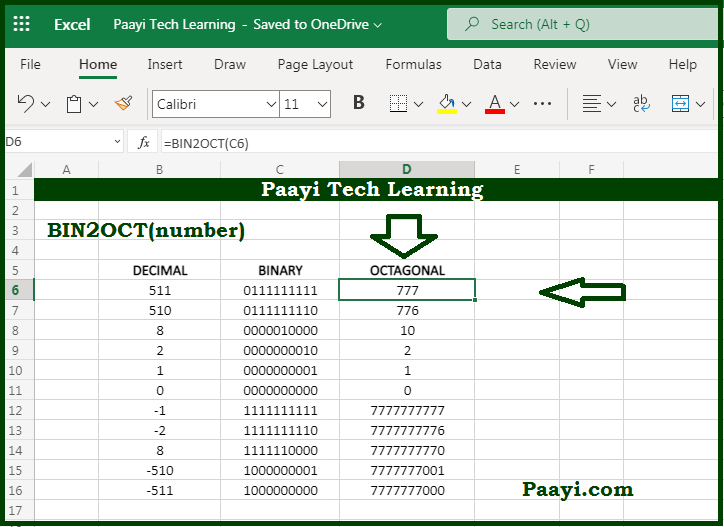# Learn How to Use Microsoft Excel BIN2OCT Function

Written by | 0 Comments | 466 Views

In this article, you will learn how to use the Microsoft Excel BIN2OCT function and its prime function in Microsoft Excel. You will also get to know the Microsoft Excel BIN2OCT function return value and syntax with the help of some examples.

Microsoft Excel BIN2OCT Function

The primary function of Microsoft Excel BIN2OCT function is used to convert binary numbers into octal. So, with the help of the BIN2OCT function, you can able to convert a binary number to its octal equivalent. The input numbers should contain zeroes and ones and be less than ten characters long; otherwise, the BIN2OCT function will return the #NUM! Error value.

Return Value of BIN2OCT Function

The return value will be the octal equivalent of the binary number.

Syntax of BIN2OCT Function

=BIN2OCT(number, [places])

Where the argument:

number: It is the binary numbers you want to convert to octal.

places: In case removed, uses the least number of characters needed to represent the number, otherwise patch the result with zeros up to the specified number of places (optional).

## How to Use Microsoft Excel BIN2OCT Function?The first bit of binary number represents whether the number is negative or positive, while the other nine digits represent the number's size. The maximum can be 511 (2^9 - 1). A negative binary number is expected to use the two's complement notation.